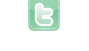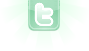## Best way to use a text displayer with time ?

Hello, I'm trying to create a new system of text displaying for my game. It has to be a small text box image that displays the text I want. The text would stay on screen for 100 time units, then the player can press "enter" to jump to the next text. At time = 0, the player can press "enter" to delete the text box. I've been testing a few things, and the best I've come out with is this :
```import greenfoot.*;
public class OverlayText extends Actor
{
public String text = "text 1";
public String text2 = "text 2";
public String text3 = "text 3";
public String text4 = "text 4";
public String text5 = "text 5";
public int time = 500;
public void act()
{
time--;
if(time == 499)
getWorld().showText(text5, getX(), getY());
if(time == 399 && Greenfoot.isKeyDown("enter"))
getWorld().showText(text4, getX(), getY());
else if(time == 399)
time++;
if(time == 299 && Greenfoot.isKeyDown("enter"))
getWorld().showText(text3, getX(), getY());
else if(time == 299)
time++;
if(time == 199 && Greenfoot.isKeyDown("enter"))
getWorld().showText(text2, getX(), getY());
else if(time == 199)
time++;
if(time == 99 && Greenfoot.isKeyDown("enter"))
getWorld().showText(text, getX(), getY());
else if(time == 99)
time++;
if(time == 0 && Greenfoot.isKeyDown("enter"))
{
getWorld().showText(text, getX(), getY());
getWorld().removeObject(this);
}
else if(time == 0)
time++;
}
}
```
What can I do to make it better ? I think there's too much code for a simple thing here.
Also, I just want to say that the OverlayText class will probably have several methods, for example if the player is given an item, etc... (and most of the code will therefore not be in the act method), so the solution must be adaptable and not be only working for this specific case with 5 String variables and a time of 500
Put String objects in an array and introduce an int index pointer. Run timer from 100 to 0 multiple times. Use image of actor to display text: To make class reusable, bring text lines in by way of a parameter of the constructor. The constructor can also initialize the image to first line of text. Like this:
```import greenfoot.*;

public class OverlayText extends Actor;
{
String[] lines;
int index;
int timer;

public OverlayText(String[] array)
{
lines = array;
showLine();
}

private void showLine()
{
if (index == lines.length)
{
getWorld().removeObject(this);
return;
}
setImage(new GreenfootImage(lines[index], 24, Color.BLACK, new Color(0, 0, 0, 0)));
timer = 100;
}

public void act()
{
if ((timer == 0 || --timer == 0) && Greenfoot.isKeyDown("enter"))
{
index++;
showLine();
}
}
}```
to be instantiate like this example:
```String[] lines =
{
"text 1",
"text 2",
"text 3",
"text 4",
"text 5"
};